Courses

# NCERT Solutions(Part - 2) - Mensuration Class 6 Notes | EduRev

## Class 6 : NCERT Solutions(Part - 2) - Mensuration Class 6 Notes | EduRev

The document NCERT Solutions(Part - 2) - Mensuration Class 6 Notes | EduRev is a part of the Class 6 Course Mathematics (Maths) Class 6.
All you need of Class 6 at this link: Class 6

Exercise 10.2

Question 1

Find the areas of the following figures by counting square:

(a)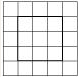(b)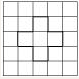(c)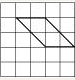(d)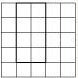(e)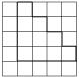(f)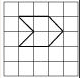(g)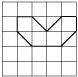(h)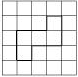(i)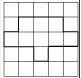(j)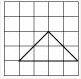(k)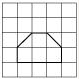(l)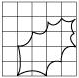(m)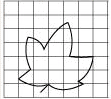(n)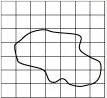(a) Number of filled square = 9

∴ Area covered by squares = 9 x 1 = 9 sq. units

(b) Number of filled squares = 5

∴ Area covered by filled squares = 5 x 1 = 5 sq. units

(c) Number of full filled squares = 2

Number of half-filled squares = 4

∴ Area covered by full filled squares = 2 x 1 = 2 sq. units

And Area covered by half-filled squares  =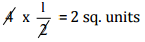∴ Total area = 2 + 2 = 4 sq. units

(d) Number of filled squares = 8

∴ Area covered by filled squares = 8 x 1 = 8 sq. units

(e) Number of filled squares = 10

∴ Area covered by filled squares = 10 x 1 = 10 sq. units

(f) Number of full filled squares = 2

Number of half-filled squares = 4

∴ Area covered by full filled squares = 2 x 1 = 2 sq. units

And Area covered by half-filled squares  =∴ Total area = 2 + 2 = 4 sq. units

(g) Number of full filled squares = 4

Number of half-filled squares = 4

∴ Area covered by full filled squares = 4 x 1 = 4 sq. units

And Area covered by half-filled squares  =∴ Total area = 4 + 2 = 6 sq. units

(h) Number of filled squares = 5

∴ Area covered by filled squares = 5 x 1 = 5 sq. units

(i) Number of filled squares = 9

∴ Area covered by filled squares = 9 x 1 = 9 sq. units

(j) Number of full filled squares = 2

Number of half-filled squares = 4

∴ Area covered by full filled squares = 2 x 1 = 2 sq. units

And Area covered by half-filled squares  =∴ Total area = 2 + 2 = 4 sq. units

(k) Number of full filled squares = 4

Number of half-filled squares = 2

∴ Area covered by full filled squares = 4 x 1 = 4 sq. units

And Area covered by half-filled squares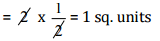∴ Total area = 4 + 1 = 5 sq. units

(l) Number of full filled squares = 3

Number of half-filled squares = 10

∴ Area covered by full filled squares = 3 x 1 = 3 sq. units

And Area covered by half-filled squares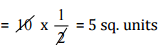∴ Total area = 3 + 5 = 8 sq. units

(m) Number of full filled squares = 7

Number of half-filled squares = 14

∴ Area covered by full filled squares = 7 x 1 = 7 sq. units

And Area covered by half-filled squares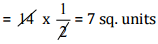∴ Total area = 7 + 7 = 14 sq. units

(n) Number of full filled squares = 10

Number of half-filled squares = 16

∴ Area covered by full filled squares = 10 x 1 = 10 sq. units

And Area covered by half-filled squares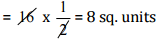∴ Total area = 10 + 8 = 18 sq. units

Exercise 10.3

Question 1

Find the areas of the rectangles whose sides are:

(a) 3 cm and 4 cm

(b) 12 m and 21 m

(c) 2 km and 3 km

(d) 2 m and 70 cm

(a) Area of rectangle = length x breadth

= 3 cm x 4 cm = 12 cm

(b) Area of rectangle = length x breadth

= 12 m x 21 m = 252 m

(c) Area of rectangle = length x breadth

= 2 km x 3 km = 6 km

(d) Area of rectangle = length x breadth

= 2 m x 70 cm

= 2 m x 0.7 m = 1.4 m

Question 2

Find the areas of the squares whose sides are:

(a) 10 cm

(b) 14 cm

(c) 5 m

Answer 2: (a) Area of square = side x side = 10 cm x 10 cm = 100 cm2

(b) Area of square = side x side = 14 cm x 14 cm = 196 cm2

(c) Area of square = side x side = 5 m x 5 m = 25 m2

Question 3

The length and breadth of three rectangles are as given below:

(a) 9 m and 6 m

(b) 17 m and 3 m

(c) 4 m and 14 m

Which one has the largest area and which one has the smallest?

Answer 3: (a) Area of rectangle = length x breadth = 9 m x 6 m = 54 m

(b) Area of rectangle = length x breadth= 3 m x 17 m = 51 m

(c) Area of rectangle = length x breadth= 4 m x 14 m = 56 m

Thus, the rectangle (c) has largest area, and rectangle (b) has smallest area.

Question 4

The area of a rectangular garden 50 m long is 300 sq m. Find the width of the garden.

Length of rectangle = 50 m and Area of rectangle = 300 m2

Since, Area of rectangle = length x breadth

Therefore,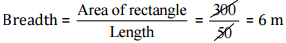Thus, the breadth of the garden is 6 m.

Question 5

What is the cost of tiling a rectangular plot of land 500 m long and 200 m wide at the rate of Rs 8 per hundred sq m?

Answer 5: Length of land = 500 m and Breadth of land = 200 m

Area of land = length x breadth = 500 m x 200 m = 1,00,000 m2

Cost of tilling 100 sq. m of land = 8

∴ Cost of tilling 1,00,000 sq. m of land =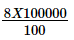=  8000

Question 6

A table-top measures 2 m by 1 m 50 cm. What is its area in square metres?

Answer 6: Length of table = 2 m

Breadth of table = 1 m 50 cm = 1.50 m

Area of table = length x breadth

= 2 m x 1.50 m = 3 m2

Question 7

A room is 4 m long and 3 m 50 cm wide. How many square metres of carpet is needed to cover the floor of the room?

Answer 7: Length of room = 4 m

Breadth of room = 3 m 50 cm = 3.50 m

Area of carpet = length x breadth

= 4 x 3.50 = 14m2

Question 8:

A floor is 5 m long and 4 m wide. A square carpet of sides 3 m is laid on the floor. Find the area of the floor that is not carpeted.

Answer 8: Length of floor = 5 m and breadth of floor = 4 m

Area of floor = length x breadth

= 5 m x 4 m = 20 m2 Now, Side of square carpet = 3 m

Area of square carpet = side x side = 3 x 3 = 9 m2

Area of floor that is not carpeted = 20 m2 – 9 m2 = 11 m2

Question 9:

Five square flower beds each of sides 1 m are dug on a piece of land 5 m long and 4 m wide. What is the area of the remaining part of the land?

Answer 9: Side of square bed = 1 m

Area of square bed = side x side = 1 m x 1 m = 1 m2

∴ Area of 5 square beds = 1 x 5 = 5 m2

Now, Length of land = 5 m

Breadth of land = 4 m

∴ Area of land = length x breadth = 5 m x 4 m = 20 m2

Area of remaining part = Area of land – Area of 5 flower beds

= 20 m2 – 5 m2 = 15 m2

Question 10

By splitting the following figures into rectangles, find their areas (The measures are given in centimetres).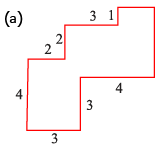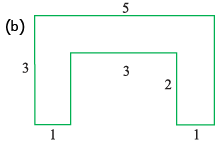(a)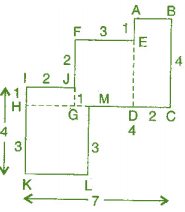Area of HKLM = 3 x 3 = 9 cm2

Area of IJGH = 1 x 2 = 2 cm2

Area of FEDG = 3 x 3 = 9 cm

Area of ABCD = 2 x 4 = 8 cm2

Total area of the figure = 9 + 2 + 9 + 8 = 28 cm

(b)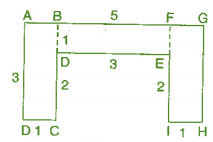Area of ABCD = 3 x 1 = 3 cm2

Area of BDEF = 3 x 1 = 3 cm2

Area of FGHI = 3 x 1 = 3 cm2

Total area of the figure = 3 + 3 + 3 = 9 cm2

Question 11

Split the following shapes into rectangles and find their areas. (The measures are given in centimetres)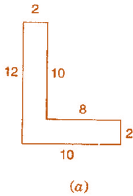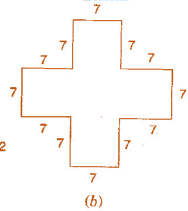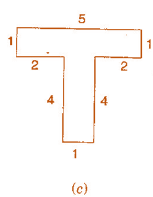(a)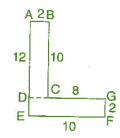Area of rectangle ABCD = 2 x 10 = 20 cm2

Area of rectangle DEFG = 10 x 2 = 20 cm2

Total area of the figure = 20 + 20 = 40 cm2

(b)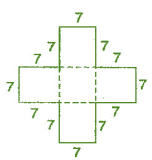There are 5 squares each of side 7 cm.

Area of one square = 7 x 7 = 49 cm

Area of 5 squares = 49 x 5 = 245 cm

(c)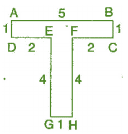Area of rectangle ABCD = 5 x 1 = 5 cm

Area of rectangle EFGH = 4 x 1 = 4 cm

Total area of the figure = 5 + 4 cm

Question 12

How many tiles whose length and breadth are 12 cm and 5 cm respectively will be needed to fit in a rectangular region whose length and breadth are respectively:

(a) 100 cm and 144 cm

(b) 70 cm and 36 cm

(a) Area of region = 100 cm x 144 cm = 14400 cm2
Area of one tile = 5 cm x 12 cm = 60 cm2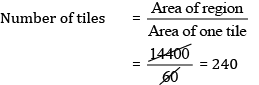Thus, 240 tiles are required.

(b) Area of region = 70 cm x 36 cm = 2520 cm2
Area of one tile = 5 cm x 12 cm = 60 cm2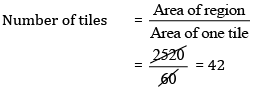Thus, 42 tiles are required.

Offer running on EduRev: Apply code STAYHOME200 to get INR 200 off on our premium plan EduRev Infinity!

## Mathematics (Maths) Class 6

185 videos|229 docs|43 tests

,

,

,

,

,

,

,

,

,

,

,

,

,

,

,

,

,

,

,

,

,

;